Maths-
General
Easy

Question

# PQ and QR are two focal chords of an ellipse and the eccentric angles of P,Q,R and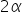,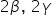respectively then tan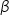tan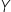is equal to -

## The correct answer is: cot2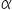###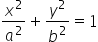P (a cos 2, b sin 2), Q (a cos 2, b sin 2)R (a cos 2, b sin 2)chord's PQ equation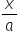cos (+) +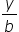sin (+) = cos (–)PQ passes through the focus (ae, 0)e =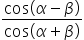PR passes through the focus (– ae, 0) the– e =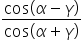= –Apply componendo and dividendo, we get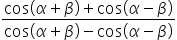=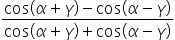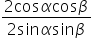=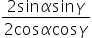tantan= cot2#### With Turito Foundation.#### Get an Expert Advice From Turito.